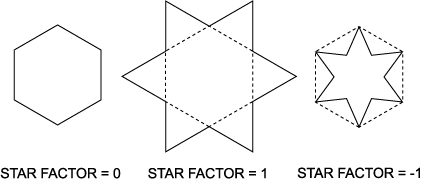### Star Factor

Star Factor causes the sides of a ring to bulge outward, or collapse inward, forming a star shape, as illustrated in the following diagram:Star Factor is normalized such that at 1, a hexagon becomes a Star of David, a square becomes a diamond, and a triangle becomes a hexagon. At Star Factor = −1, a triangle becomes the Mercedes symbol. At zero, Star Factor has no effect.

A ring is composed of two alternating sets of vertices, called even and odd. The even vertices are the "real" polygon. The odd vertices are normally hidden, but they become the points of the star when Star Factor is positive, or the inner angles when Star Factor is negative.

The odd vertices collectively form a second polygon, which can be independently rotated; see Pinwheel. Note that when Star Factor has a large negative value, the odd vertices stay visible for a long time, which may reduce performance.